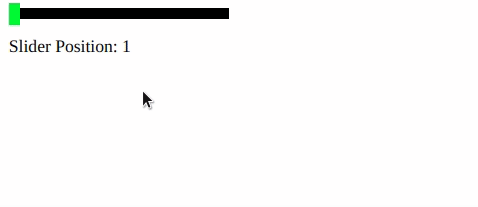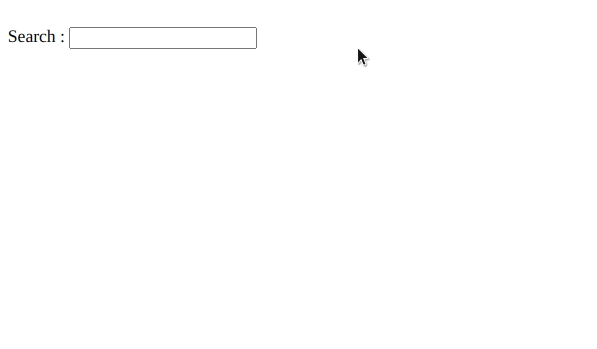Skip to content
Related Articles
script.aculo.us Modules
• Last Updated : 20 Nov, 2020

script.aculo.us extends the Prototype JavaScript Framework by adding more accessibility features to your webpage. These features are divided into several modules.

1. Effects Module: The effects’ module comes with five core effects : Opacity, Scale, MoveBy, Highlight, and Parallel. Through the combination of these core effects there are over 16 additional effects eg: effect.Appear, effect.Toggle etc.

Example:

## HTML

 ```<``html``>`` ` `<``head``>``    ``<``script` `type``=``"text/javascript"` `        ``src``=``"prototype.js"``>``    ````     ` `    ``<``script` `type``=``"text/javascript"` `        ``src``=``"scriptaculous.js?load = effects"``>``    ````     ` `    ``<``script` `type``=``"text/javascript"``>``        ``function ShowElement(element) {``            ``new Effect.Opacity(element, ``            ``{ duration: 1, from: 0, to: 1.0 });``        ``}`` ` `        ``function HideElement(element) {``            ``new Effect.Opacity(element, ``            ``{ duration: 1, from: 1.0, to: 0 });``        ``}``    `````` ` `<``body``>``    ``<``div` `onclick``=``"ShowElement('element')"``>``        ``<``Button``>Show Content``    ````    ``<``br` `/>`` ` `    ``<``div` `onclick``=``"HideElement('element')"``>``        ``<``Button``>Hide Content``    ````    ``<``br` `/>``    ``<``img` `id``=``"element"` `src``=``"./gfg.png"``>```` ` ``

Output:2. Drag and Drop: It can be used to make any element draggable, turn it into a drop zone, or even make an entire series of elements sortable so that you can rearrange them by dragging and dropping.

Example:

## HTML

 ```<``html``>`` ` `<``head``>``    ``<``script` `type``=``"text/javascript"` `        ``src``=``"prototype.js"``>``    ````     ` `    ``<``script` `type``=``"text/javascript"` `        ``src``=``"scriptaculous.js?load=effects,dragdrop"``>``    ````     ` `    ``<``script` `type``=``"text/javascript"``>``        ``var dragables = ['element'];``        ``window.onload = function () {``            ``dragables.each(function (item) ``            ``{ new Draggable(item, {}); });``        ``}            ``    `````` ` `<``body``>``    ``<``img` `id``=``"element"` `src``=``"./gfg.png"``>```` ` ``

Output:3. Sliders: A slider is a kind of small track, along which you can slide a handle. It translates into a numerical value. With script.aculo.us, you can create such sliders with plenty of control.

Example:

## HTML

 ```<``html``>`` ` `<``head``>``    ``<``script` `type``=``"text/javascript"` `        ``src``=``"prototype.js"``>``    ````     ` `    ``<``script` `type``=``"text/javascript"` `        ``src``=``"scriptaculous.js?load = slider"``>``    ````     ` `    ``<``script``>``        ``window.onload = function () {``            ``new Control.Slider('handle', 'track', {``                ``range: \$R(1, 100),``                ``values: [1, 10, 20, 30, 40, ``                    ``50, 60, 70, 80, 90, 100],``                ``sliderValue: 1,``                ``onSlide: function (value) {``                    ``\$('sliderValue').innerHTML ``                        ``= 'Slider Position: ' + value;``                ``}``            ``});``        ``}``    ```` ` `    ``<``style``>``        ``.track {``            ``background-color: rgb(0, 0, 0);``            ``position: relative;``            ``height: 10px;``            ``width: 200px;``            ``cursor: pointer;``        ``}`` ` `        ``.handle {``            ``background-color: #13e421;``            ``height: 20px;``            ``width: 4.25px;``            ``top: -4.25px;``            ``cursor: move;``        ``}``    `````` ` `<``body``>``    ``<``div` `id``=``"track"` `class``=``"track "``>``        ``<``div` `id``=``"handle"` `class``=``"handle"` `            ``style``=``"width: 10px;"``>``        ````    ```` ` `    ``<``p` `id``=``"sliderValue"``>```` ` ``

Output:4. Autocompletion: Autocompletion allows local and server-powered autocompleting text input fields. It makes autocompletion very easy.

Example:

## HTML

 ```<``html``>`` ` `<``head``>``    ``<``script` `type``=``"text/javascript"` `        ``src``=``"prototype.js"``>``    ````     ` `    ``<``script` `type``=``"text/javascript"` `        ``src``=``"scriptaculous.js?load = effects,controls"``>``    ````     ` `    ``<``script` `type``=``"text/javascript"``>``        ``window.onload = function () {``            ``new Autocompleter.Local(``                ``'searchBox',``                ``'Result',``                ``['effect', 'drag', 'drop', ``                ``'auto', 'complete', ``                ``'slider', 'sound'],``            ``);``        ``}``    `````` ` `<``body``>``    ``<``div``>``        ``<``label``>Search :``        ``<``input` `type``=``"text"` `id``=``"searchBox"` `/>``        ``<``div` `id``=``"Result"``>``    `````` ` ``

Output:5. In-place Editing: It allows to make any text or collection of items in the database, editable in-place by simply clicking on it. You can turn the static content to editable form using this module.

6. Builder: Builder allows creating DOM elements dynamically. This tool eases the DOM creation.

7. Sound: This module was first introduced in version 1.7.1 of the script. It lets you play sounds easily, queue them up, use multiple tracks, and so on.< It helps to easily manage sound tracks.

My Personal Notes arrow_drop_up